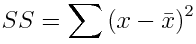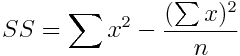The sum of squares of a data set is the sum of the squared deviations from the mean. The conceptual formula for the sum of squares is:An equivalent and more computationally convenient formula is:The sum of squares is not often reported as a descriptive statistic, but it is used extensively in statistical calculations. For example, it is used to calculate the variance and the standard deviation (to follow). It is also used extensively in analysis of variance and regression analysis. Return To Main Page